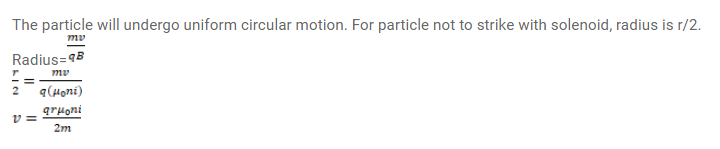# A tightly-wound, long solenoid has n turns per unit length,

Question:

A tightly-wound, long solenoid has $\mathrm{n}$ turns per unit length, a radius $\mathrm{r}$ and carries a current $\mathrm{i}$. A particle having charge $q$ and mass $m$ is projected from a point on the axis in a direction perpendicular to the axis. What can be the maximum speed for which the particle does not strike the solenoid?

Solution: